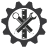SuperTeacherTools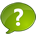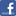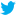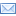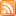# Join Code:NPUEB2

How to Play:

Instant Jeopardy Review is designed for live play with as many individuals or teams as you like! Each team will need to enter the Join Code above. Teams choose a question, then try to give the best answer.

Scoring is built in for each team.

You can also choose to use a timer below.

Have fun!

Play This Game Live Now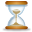Seconds To Answer Each Question
Set to X to hide the timer

Prefer the old Flash template? Switch now: 6th Grade Math Jeopardy Jeopardy Review Flash Version

6th Grade Math Jeopardy

 Order of Operations Decimals Fractions Multiples and Factors Area and Perimeter 100 100 100 100 100 200 200 200 200 200 300 300 300 300 300 400 400 400 400 400 500 500 500 500 500 Final Question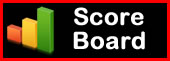Evaluate the expression:  100 - 80 ÷ 4 + 3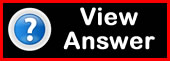Evaluate the expression: 52 × 4 ÷ 2 - (8-3)Here is how a student simplified an expression.  Is this correct? If not, where did the student amek a mistake?

2 × (12-3)2 +7

2 × (12-9) + 7

2 × 3 + 7

6 + 7

13Evaluate the following expression:

7.68 + 2.24Evaluate the Expression:

2 1/10 × 3 1/6In a parade, 18 boys and 24 girls are merching in seperate rows.  Each rwo requires the same number of students.  What is the greatest number of students that can be in a row?What is the perimeter of a rectangle with width of 25 m and a heigth of 12 m?What is the area of a a Parallelogram with base of 15 yd and a height of 12 yd?What is the area of a triangle witha base of 5 in and a heigth of 3 in?

A =  1/2 bhFind the area of a triangle owith heigth of 27 cm and a base of 25 cm.

A = 1/2 bhFind the circumference of a circle with a radius of 5 in.

C = 2∏ rThe Answer is:  126

The answer is:  83

The answer is 69

The answer is:  45

Answer:

The student did not subtract 12 - 3 before squaring.  It should look like this:

2 × (12-3)2 + 7

2 × 92 + 7

2 × 81 + 7

162 + 7

169

Answer is:  9.92

The answer is:  35.4

The answer is:  2.940

The answer is:

7.5

The answer is:

61.1

The answer is:

1/2

The answer is:

4 4/5

The Answer is:

6 39/60

The Answer is:

16/35

The Answer is:

7/40

The Answer is:

1,2,4,8,16,32,64

Answer:

16, 32, 48, 64, 80

Answer:

84 - 1,2,3,4,6,7,12,14,21,28,42,84

120 - 1,2,3,4,5,6,8,10,12,15,20,24,30,40,60,120

GCF is 12

Answer:

5- 5,10,15,20,25,30,35,40,45,50

6, - 6,12,18,24,30,36,42,48

10 - 10,20,30,40,50

LCM is 30

Answer:

Look for the GCF

18 - 1,2,3,6,9,18

24 - 1,2,3,4,6,8,12,24

The GCF is 6.  Therefore the greatest number of students that can be in a row is 6.

Answer: 74 m

Answer:

Area is 180 yd2

Answer:

7.5 in22

Answer:

A = 337.5 cm

Answer:  31.4 in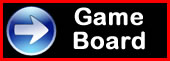Remove Teams / Players